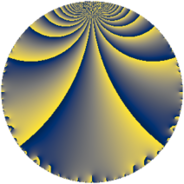# Properties

 Label 169.4.cLevel $169$ Weight $4$ Character orbit 169.c Rep. character $\chi_{169}(22,\cdot)$ Character field $\Q(\zeta_{3})$ Dimension $66$ Newform subspaces $12$ Sturm bound $60$ Trace bound $3$

# Related objects

## Defining parameters

 Level: $$N$$ $$=$$ $$169 = 13^{2}$$ Weight: $$k$$ $$=$$ $$4$$ Character orbit: $$[\chi]$$ $$=$$ 169.c (of order $$3$$ and degree $$2$$) Character conductor: $$\operatorname{cond}(\chi)$$ $$=$$ $$13$$ Character field: $$\Q(\zeta_{3})$$ Newform subspaces: $$12$$ Sturm bound: $$60$$ Trace bound: $$3$$ Distinguishing $$T_p$$: $$2$$

## Dimensions

The following table gives the dimensions of various subspaces of $$M_{4}(169, [\chi])$$.

Total New Old
Modular forms 106 86 20
Cusp forms 78 66 12
Eisenstein series 28 20 8

## Trace form

 $$66 q - q^{2} + 7 q^{3} - 107 q^{4} - 4 q^{5} - 30 q^{6} + 35 q^{7} + 30 q^{8} - 204 q^{9} + O(q^{10})$$ $$66 q - q^{2} + 7 q^{3} - 107 q^{4} - 4 q^{5} - 30 q^{6} + 35 q^{7} + 30 q^{8} - 204 q^{9} + 99 q^{10} - 15 q^{11} - 336 q^{12} - 132 q^{14} + 124 q^{15} - 331 q^{16} + 59 q^{17} + 614 q^{18} - 111 q^{19} + 311 q^{20} - 206 q^{21} - 434 q^{22} + 267 q^{23} - 216 q^{24} + 58 q^{25} - 770 q^{27} + 240 q^{28} + 169 q^{29} - 28 q^{30} + 428 q^{31} - 151 q^{32} + 361 q^{33} + 182 q^{34} + 236 q^{35} + 675 q^{36} - 417 q^{37} - 1192 q^{38} + 418 q^{40} + 373 q^{41} - 172 q^{42} + 439 q^{43} - 848 q^{44} - 16 q^{45} + 230 q^{46} + 204 q^{47} + 42 q^{48} - 506 q^{49} + 1106 q^{50} + 770 q^{51} - 1616 q^{53} - 1314 q^{54} + 14 q^{55} - 86 q^{56} + 330 q^{57} + 193 q^{58} - 1673 q^{59} - 144 q^{60} + 845 q^{61} - 1190 q^{62} - 70 q^{63} + 2714 q^{64} + 5064 q^{66} + 387 q^{67} + 441 q^{68} + 53 q^{69} - 2560 q^{70} + 781 q^{71} - 1155 q^{72} - 1600 q^{73} + 395 q^{74} - 2083 q^{75} + 100 q^{76} + 674 q^{77} - 32 q^{79} - 2153 q^{80} + 2099 q^{81} - 2637 q^{82} - 1776 q^{83} + 1540 q^{84} - 1426 q^{85} + 5172 q^{86} + 2841 q^{87} - 828 q^{88} + 655 q^{89} + 1138 q^{90} + 2408 q^{92} + 2052 q^{93} + 1156 q^{94} + 2136 q^{95} - 2816 q^{96} - 177 q^{97} + 1513 q^{98} - 5892 q^{99} + O(q^{100})$$

## Decomposition of $$S_{4}^{\mathrm{new}}(169, [\chi])$$ into newform subspaces

Label Dim $A$ Field CM Traces $q$-expansion
$a_{2}$ $a_{3}$ $a_{5}$ $a_{7}$
169.4.c.a $2$ $9.971$ $$\Q(\sqrt{-3})$$ None $$-5$$ $$7$$ $$14$$ $$-13$$ $$q+(-5+5\zeta_{6})q^{2}+(7-7\zeta_{6})q^{3}-17\zeta_{6}q^{4}+\cdots$$
169.4.c.b $2$ $9.971$ $$\Q(\sqrt{-3})$$ None $$-3$$ $$1$$ $$-18$$ $$-15$$ $$q+(-3+3\zeta_{6})q^{2}+(1-\zeta_{6})q^{3}-\zeta_{6}q^{4}+\cdots$$
169.4.c.c $2$ $9.971$ $$\Q(\sqrt{-3})$$ None $$3$$ $$1$$ $$18$$ $$15$$ $$q+(3-3\zeta_{6})q^{2}+(1-\zeta_{6})q^{3}-\zeta_{6}q^{4}+\cdots$$
169.4.c.d $2$ $9.971$ $$\Q(\sqrt{-3})$$ None $$4$$ $$-2$$ $$-34$$ $$20$$ $$q+(4-4\zeta_{6})q^{2}+(-2+2\zeta_{6})q^{3}-8\zeta_{6}q^{4}+\cdots$$
169.4.c.e $2$ $9.971$ $$\Q(\sqrt{-3})$$ None $$5$$ $$7$$ $$-14$$ $$13$$ $$q+(5-5\zeta_{6})q^{2}+(7-7\zeta_{6})q^{3}-17\zeta_{6}q^{4}+\cdots$$
169.4.c.f $4$ $9.971$ $$\Q(\sqrt{-3}, \sqrt{17})$$ None $$-5$$ $$-5$$ $$30$$ $$15$$ $$q+(-2+\beta _{1}+2\beta _{2}+\beta _{3})q^{2}+(-1+\cdots)q^{3}+\cdots$$
169.4.c.g $4$ $9.971$ $$\Q(\sqrt{-3}, \sqrt{17})$$ None $$-1$$ $$-5$$ $$-6$$ $$9$$ $$q-\beta _{1}q^{2}+(3\beta _{1}-4\beta _{2})q^{3}+(4+\beta _{1}+\cdots)q^{4}+\cdots$$
169.4.c.h $4$ $9.971$ $$\Q(\zeta_{12})$$ None $$0$$ $$-4$$ $$0$$ $$0$$ $$q-\zeta_{12}^{2}q^{2}-2\zeta_{12}q^{3}+(5-5\zeta_{12}+\cdots)q^{4}+\cdots$$
169.4.c.i $4$ $9.971$ $$\Q(\zeta_{12})$$ None $$0$$ $$14$$ $$0$$ $$0$$ $$q-2\zeta_{12}^{2}q^{2}+7\zeta_{12}q^{3}+(-4+4\zeta_{12}+\cdots)q^{4}+\cdots$$
169.4.c.j $4$ $9.971$ $$\Q(\sqrt{-3}, \sqrt{17})$$ None $$1$$ $$-5$$ $$6$$ $$-9$$ $$q+\beta _{1}q^{2}+(3\beta _{1}-4\beta _{2})q^{3}+(4+\beta _{1}+\cdots)q^{4}+\cdots$$
169.4.c.k $18$ $9.971$ $$\mathbb{Q}[x]/(x^{18} - \cdots)$$ None $$-5$$ $$-1$$ $$60$$ $$-38$$ $$q+(-\beta _{1}+\beta _{2}-\beta _{3})q^{2}+(\beta _{11}-\beta _{14}+\cdots)q^{3}+\cdots$$
169.4.c.l $18$ $9.971$ $$\mathbb{Q}[x]/(x^{18} - \cdots)$$ None $$5$$ $$-1$$ $$-60$$ $$38$$ $$q+(\beta _{1}-\beta _{2}+\beta _{3})q^{2}+(\beta _{11}-\beta _{14})q^{3}+\cdots$$

## Decomposition of $$S_{4}^{\mathrm{old}}(169, [\chi])$$ into lower level spaces

$$S_{4}^{\mathrm{old}}(169, [\chi]) \cong$$ $$S_{4}^{\mathrm{new}}(13, [\chi])$$$$^{\oplus 2}$$